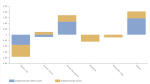# DCPY.ROBUSTSCALER(with_centering, with_scaling, quantile_range_min, quantile_range_max, column)

The Robust Scaler scales data according to the interquartile range and removes the median. It is a better alternative than the Standard Scaler (removing the mean and scaling to unit variance) in case of a higher number of outliers.

###### Parameters
• with_centering – Specifies if the data needs to be centered, Boolean (for example, True).
• with_scaling – Specifies if the data needs to be scaled to the interquartile range, Boolean (for example, True).
• quantile_range_min – Lower bound of the IQR used for scaling, float (for example, 25).
• quantile_range_max – Upper bound of the IQR used for scaling, float (for example, 75).
• columns – Dataset column or custom calculation.

Example: DCPY.ROBUSTSCALER(True, True, 25, 75, [Discount])

###### Input data
• Numeric column.
• Rows containing missing values are dropped before calculations.
###### Result
• A numeric column with transformed values with the same length as the input column.
• Missing values are on the same indices as in the input column.
###### Key usage points
• Use it when the data contains a large number of outliers.
###### Example

The following example shows how the car weight and fuel economy (mpg) are scaled using the following functions:

• DCPY.ROBUSTSCALER(True, True, 25, 75, [MPG])

• DCPY.ROBUSTSCALER(True, True, 25, 75, [WT])

The two scaled values are visualized in the Butterfly visualization.For the whole list of algorithms, see Data science built-in algorithms.